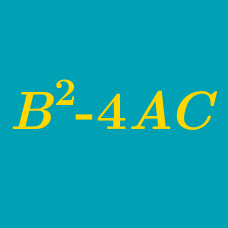Algebra

# Quadratic Discriminant: Level 4 Challenges

What is the sum of all the integers $a$ such that the following equation has no real roots:

$\frac{x^2+x+a-5}{x-1}=a?$

$k$ is uniformly chosen from the interval $[ -5, 5]$. Let $p$ be the probability that the quadratic $f(x) = x^2 + kx + 1$ has both roots between -2 and 4. What is the value of $\lfloor 1000 p \rfloor$?

Details and assumptions

Greatest Integer Function: $\lfloor x \rfloor: \mathbb{R} \rightarrow \mathbb{Z}$ refers to the greatest integer less than or equal to $x$. For example $\lfloor 2.3 \rfloor = 2$ and $\lfloor -3.4 \rfloor = -4$.

Find the sum of integral values of $\alpha$ such that $2\log (x+3)=\log (\alpha x)$ has exactly one real solution and $|\alpha |<20$.

If $\alpha$ is one of the non-real seventh roots of unity, then find the discriminant of the monic quadratic equation with the roots $\alpha+\alpha^2+\alpha^4$ and $\alpha^3+\alpha^5+\alpha^6.$

Details and Assumptions:

• The discriminant of a quadratic equation $ax^2+bx+c=0$ is $b^2-4ac.$

$(a-1)(x^{2}+x+1)^{2}-(a+1)(x^{4}+x^{2}+1)=0$

Provided that two roots of the above equation are real and distinct for $a \in \mathbb{R}-A,$ find the set $A.$

×